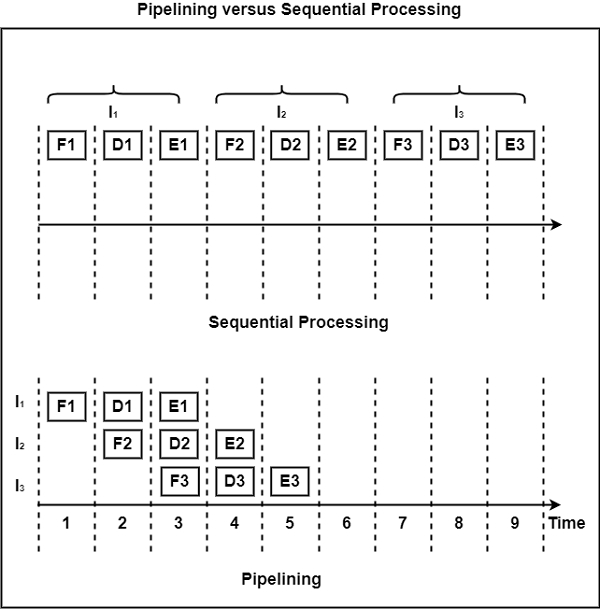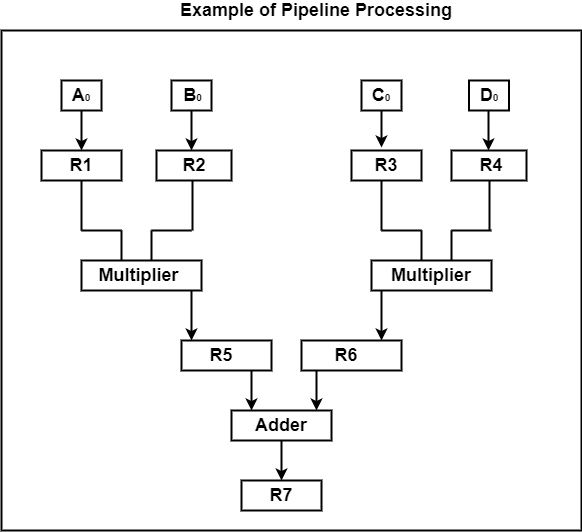# What is the process of pipelining?

Pipelining is a technique of breaking a sequential process into small fragments or sub-operations. The execution of each of these sub-procedure takes place in a certain dedicated segment that functions together with all other segments. The pipeline has a collection of processing segments which helps the flow of binary information.

The internal working in a pipeline is such that the outcome of one segment is conveyed to the next segment in the pipeline until the desired result is obtained. The outcome is acquired after the information is developed through all segments.

The term “pipeline” indicates that the flow of information takes place in parallel. Pipelining defines the temporal overlapping of processing. The overlapping of processing is done by relating a register with every segment in the pipeline. The registers help in providing isolation between each segment so that every segment can work on distinct data simultaneously.

A segment consists of an input register and a combinational circuit. The register stores the information and the combinational circuit operates in the specific segment. The output of the combinational circuit of a segment is sent to the input register of the next segment. To perform the activity in each segment, a clock is set for each register.

When we consider a sequential execution of three instructions, each having three stages of execution, the sequence got is the following instruction cycle.

If each stage requires one unit time and a separate unit for each action, then the total time taken would be nine units. In the case of pipelined execution of the same instruction set, the sequence would require only five units.

The figure shows the comparison between parallel processing and sequential processing.As observed in the figure, we can save approximately 50% of the execution time by using pipelining.

The pipeline organization will be indicated using a simple example. Suppose that we need to execute the combined multiply and add operations with a cascade of numbers.

Ai * Bi + Ci for i = 1,2,3 … 7

Each sub-operation is executed in a segment inside a pipeline. The separation between each segment is provided by registers for each segment to work on different data simultaneously.

Each segment uses one or three registers with combinational circuits. R1 through R7 are registers that get new information with each clock pulse. The combinational circuits are multiplier and adder.

R1 ← Ai, R1 ← Bi Input Ai and Bi

R3 ← R1 * R2, R4 ← C Multiply and Input Ci

R5 ← R3 + R4 Add Ci to product